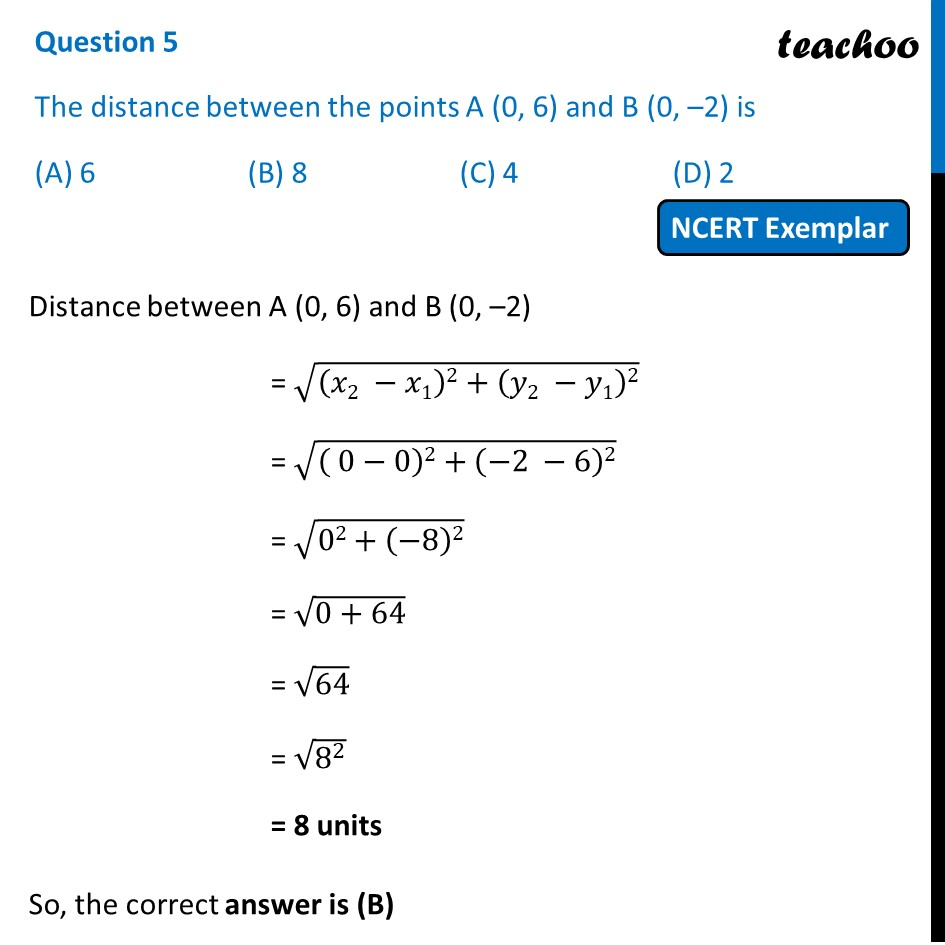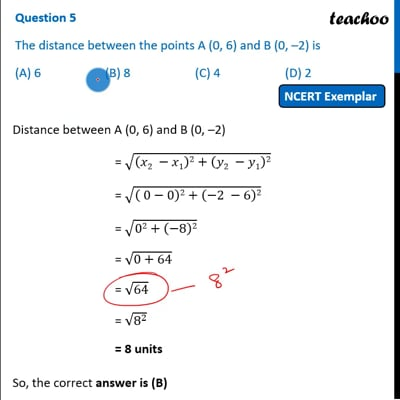NCERT Exemplar - MCQ

Chapter 7 Class 10 Coordinate Geometry
Serial order wise

## (A) 6   (B) 8   (C) 4   (D) 2This video is only available for Teachoo black users

Introducing your new favourite teacher - Teachoo Black, at only ₹83 per month

### Transcript

Question 5 The distance between the points A (0, 6) and B (0, –2) is (A) 6 (B) 8 (C) 4 (D) 2 Distance between A (0, 6) and B (0, –2) = √((𝑥2 −𝑥1)2+(𝑦2 −𝑦1)2) = √(( 0−0)2+(−2 −6)2) = √(02+(−8)2) = √(0+64) = √64 = √(8^2 ) = 8 units So, the correct answer is (B)